19 篇文章 0 订阅

函数重载

C++中引入了一个新特性，函数重载。

int test(int i, int j)
{
cout << "test" << endl;
}

int test(double i, int j)
{
cout << "test" << endl;
}

int test(double i, int j, int k)
{
cout << "test" << endl;
}

为什么C++支持重载，C语言不支持呢？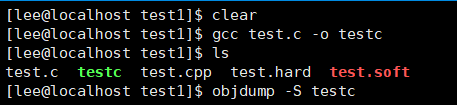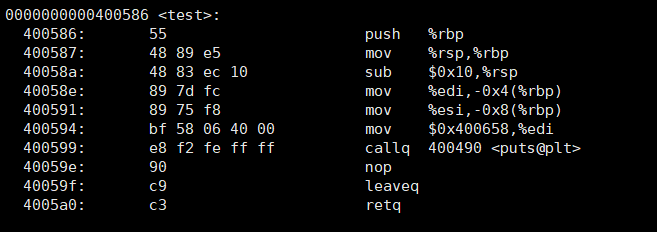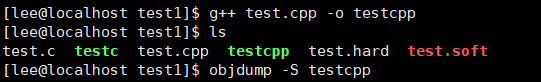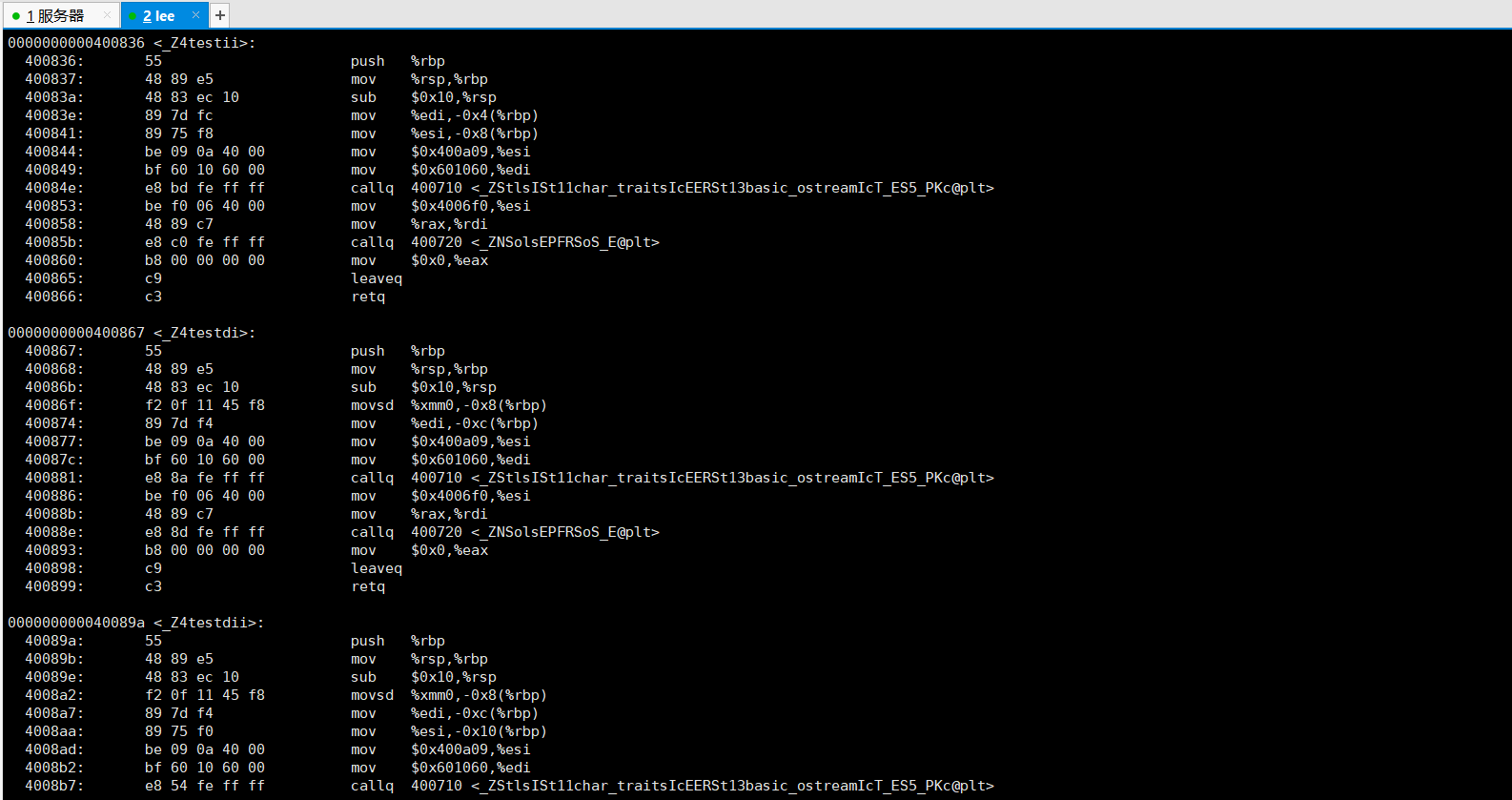_Z是所有函数的前缀，4是函数名的字符个数，例如第一个_Z4testii则代表函数名为test,具有四个字符，参数分别是ii。

extern “C”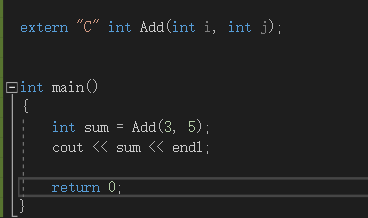引用

引用的概念

int main()
{
int i = 5;
int& j = i;
cout << i << ' ' << j << endl;
j = 8;
cout << i << ' ' << j << endl;

return 0;
}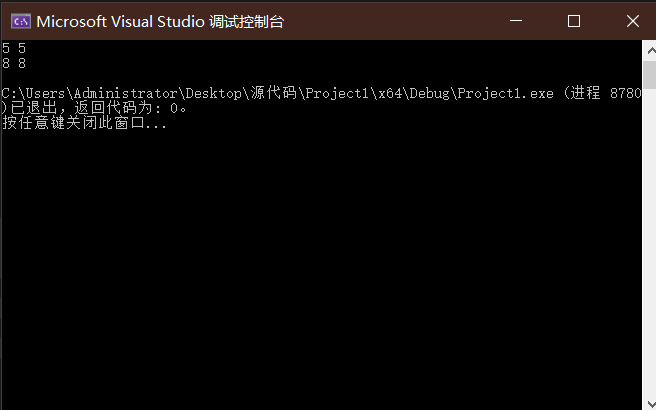引用的特性

1. 引用在定义的时候必须初始化（因为引用是某个对象的别名，所以必须初始化）
2. 一个对象可以有多个引用
3. 一旦引用一个实体，就不能再引用别的实体（有点类似指针的顶层const）

常引用

int main()

{
const int i = 5;
int& j = i;
//错误的
const int & k = i;
正确的
}

int main()
{
int x = 6;
const int & y = x;
}

引用的跨类型

int main()
{
double x = 3.14;
int& y = x;
//错误的

const int& z = x;
//正确的
return 0;
}

int main()
{
double x = 3.14;
int& y = x;
/*
等价于
const int &temp = x;
int &y = temp;
*/

const int& y = x;
/*
等价于
const int &temp = x;
const int &z = temp;
*/

return 0;
}

引用的使用场景

1. 作为参数
struct A
{
int arr;
};

void test(A& s1)
{

}

1. 作为返回值
> int& Add(int a, int b)
{
int c = a + b;
return c;
}

int main()
{
cout << ret << endl;
return 0;
}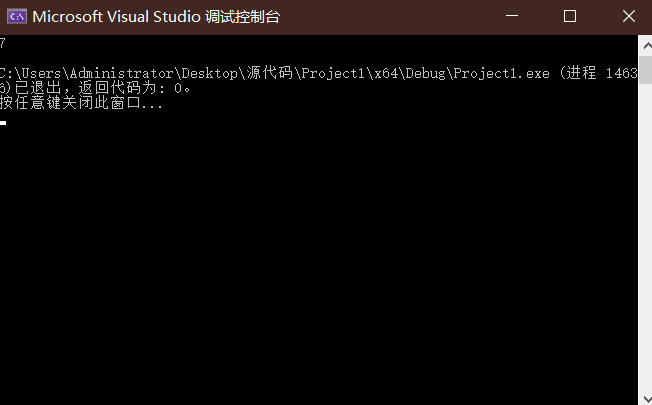引用和指针

int main()
{
int x = 5;
int& y = x;
int* z = &x;

return 0;
}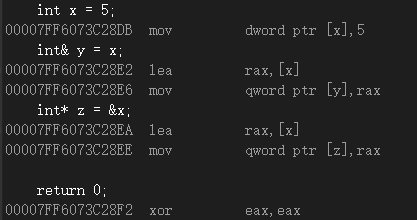引用和指针的不同点:

1. 引用在定义时必须初始化，指针没有要求
2. 引用在初始化时引用一个实体后，就不能再引用其他实体，而指针可以在任何时候指向任何一个同类型 实体
3. 没有NULL引用，但有NULL指针
4. 在sizeof中含义不同：引用结果为引用类型的大小，但指针始终是地址空间所占字节个数(32位平台下占 4个字节)
5. 引用自加即引用的实体增加1，指针自加即指针向后偏移一个类型的大小
6. 有多级指针，但是没有多级引用
7. 访问实体方式不同，指针需要显式解引用，引用编译器自己处理
8. 引用比指针使用起来相对更安全

内联函数

inline关键字修饰的函数就是内联函数，在编译时编译器会将函数的代码在调用内联函数的地方展开，减去了函数压栈的开销，提升程序运行的效率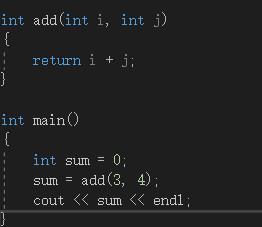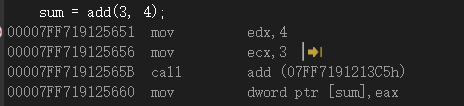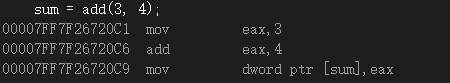内联函数的特性

• 内联函数是一种用空间换时间的做法，省去了创建栈帧和压栈的开销，但也因此代码很复杂和具有循环或递归之类的函数不适合作为内联函数，就算声明为内联函数编译器也会自动将其忽略。
• 内联函数不能声明和定义分离，因为一旦声明为内联函数，在调用的时候就会直接展开，没有了函数的地址，就无法将其链接到定义的部分。

auto

int main()
{
auto i = 2.7;
cout << i <<"的类型为：" << typeid(i).name() << endl;
}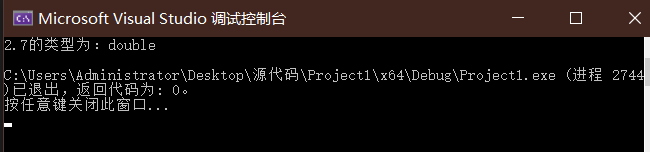auto的使用规则

1. auto可以与指针和引用结合
int main()
{
int i = 4;
auto a1 = &i;
auto *a2 = &i;
auto& a3 = i;

cout <<"a1的类型为：" << typeid(a1).name() << endl;
cout << "a2的类型为：" << typeid(a2).name() << endl;
cout << "a3的类型为：" << typeid(a3).name() << endl;
}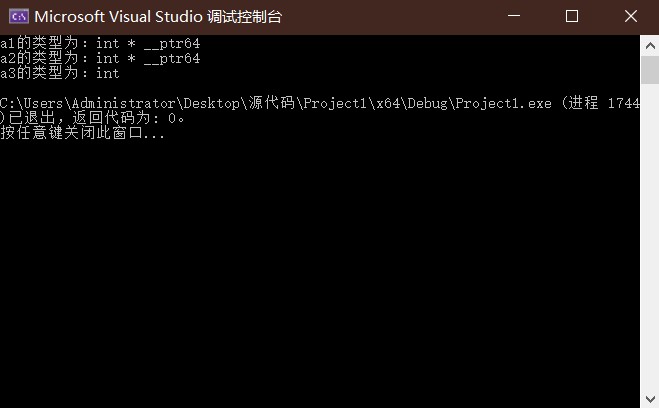1. 在同一行定义多个变量
int main()
{
auto i = 1, j = 2; //正确，同一行都是相同类型
auto x = 3, y = 4.8;//错误，同一行类型不同
}
1. auto不能作为函数的参数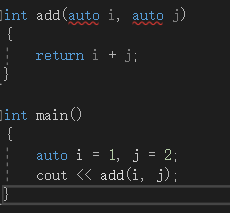auto不能作为形参的类型，编译器无法对i和j的类型进行推导。

2. auto不能直接用来声明数组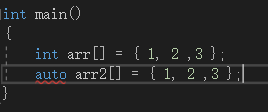3. auto一般会忽略顶层const

int main()
{
const int i = 5;
//i : const int
auto a1 = i;
//忽略了顶层const ,al : int
const auto a2 = i;
//加上const， a2 : const int
}

范围for循环

int main()
{
vector<int> vec{ 1, 3, 5, 7, 9 };

for (auto i : vec)
{
cout << i << ends;
}

}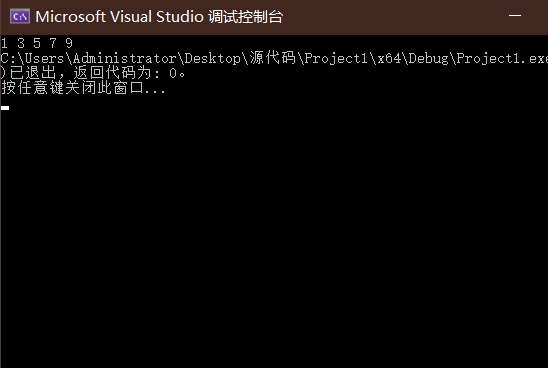06-198万+
07-16789009-051909
05-21302
12-141万+
11-13980
12-126万+
01-221473
09-031万+
01-15682
11-2048
04-181188
09-071万+
12-044021
05-308935
11-12126
12-042748
11-2336
02-12956
02-16201¥2 ¥4 ¥6 ¥10 ¥20余额支付 (余额：-- )扫码支付获取中扫码支付点击重新获取扫码支付1.余额是钱包充值的虚拟货币，按照1:1的比例进行支付金额的抵扣。
2.余额无法直接购买下载，可以购买VIP、C币套餐、付费专栏及课程。余额充值